# Wavy and the sampling or Nyquist-Shannon theorem

### The sampling theorem or Nyquist-Shannon theorem

This post deals with one of the fundamental theorem of signal processing: the sampling theorem or Nyquist-Shannon theorem (have a look on wikipedia). What is the right sampling to transform an analog signal to a digital one?First of all the news:  I decided that having a friend when I try to write about signal processing is useful. So I gave a name to my friendand the name I chose is Wavy :)…but I had a look on google for ‘wavy’ and I found many beautiful images of wavy hair..for this reason, now, Wavy becamewith some hair :). Let Wavy answer to the questions of our latest post.

#### Questions

• What happen if we use longer nsample?
• What happen if we use smaller sampling frequency?
##### First of all, let’s enunciate the sampling theorem:
If a continuous signal$\displaystyle x(t)$ is band-limited, that is its Fourier spectrum is null above a given frequency$\displaystyle F_M$, and if the sampling rate is higher than 2 times the maximum frequency$\displaystyle F_M$  of the signal, than the continuous signal can be perfectly recovered by the discrete signal$\displaystyle x(n)=x(nTs)$if$\displaystyle F_s = \frac{1}{T_s}>2 F_{M}$$\displaystyle F_s$ is the Sampling Frequency.In other words, if we want to fair digitalize a continuous signal which has as maximum frequency content$\displaystyle F_M$, we need to sample the data with a frequency at minimum equal to$\displaystyle 2 F_M$, that is peak a point each$\displaystyle T_s = \frac{1}{2F_M}$ time.At this point let’s come back to Wavy and his questions, and let’s start from second question:
• What happen if we use smaller sampling frequency?

Let’s simulate again a sinusoidal signal which has as main frequency nu=50Hz
In :
### importing the library
from __future__ import print_function
import numpy as np
import matplotlib.pyplot as plt
%matplotlib inline

from scipy.fftpack import fft
# sample spacing
sampling=1024
nsample = 500
dt = 1.0 / sampling
x = np.linspace(0.0, nsample*dt,nsample)
nu=50.0# frequency in Hz of the sine function
y = np.sin(nu * 2.0*np.pi*x)
yf = fft(y)
xf = np.linspace(0.0, 1.0/(2.0*dt), nsample/2)
plt.plot(x, (y[0:nsample]))
plt.grid()
plt.show()
plt.plot(xf, 2.0/nsample * np.abs(yf[0:nsample/2]))
plt.grid()
plt.show()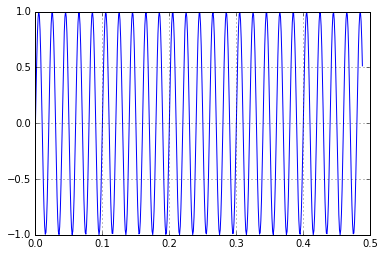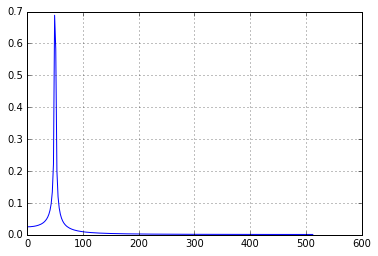We have used sampling frequency sampling=1024 Hz which is certainly $> 2*nu$. Now let’s do the same experiment using sampling=$60$Hz, which is smaller than 2 times nu
In :
# sample spacing
sampling=60
nsample = 500
dt = 1.0 / sampling
x = np.linspace(0.0, nsample*dt,nsample)
nu=50.0# frequency in Hz of the sine function
y = np.sin(nu * 2.0*np.pi*x)
yf = fft(y)
xf = np.linspace(0.0, 1.0/(2.0*dt), nsample/2)
plt.plot(x, (y[0:nsample]))
plt.grid()
plt.show()
plt.plot(xf, 2.0/nsample * np.abs(yf[0:nsample/2]))
plt.grid()
plt.show()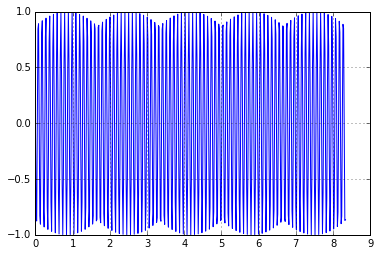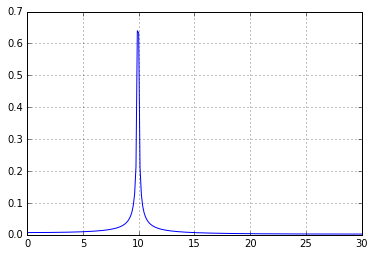The signal in time domain isn’t not certainly a single sinusoidal signal at $50$Hz, but seems a beating of 2 different frequency. The main frequency is not anymore $50$Hz, but $10$ Hz… Now let’s sample the data at $70$Hz
In :
# sample spacing
sampling=70
nsample = 500
dt = 1.0 / sampling
x = np.linspace(0.0, nsample*dt,nsample)
nu=50.0# frequency in Hz of the sine function
y = np.sin(nu * 2.0*np.pi*x)
yf = fft(y)
#xf = np.linspace(0.0, 1.0/(2.0*dt), nsample/2)
xf = np.linspace(0.0, 1.0/(2.0*dt), nsample/2)
plt.plot(x, (y[0:nsample]))
plt.grid()
plt.show()
plt.plot(xf, 2.0/nsample * np.abs(yf[0:nsample/2]))
plt.grid()
plt.show()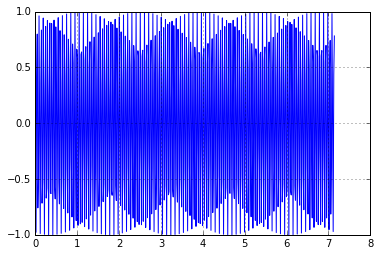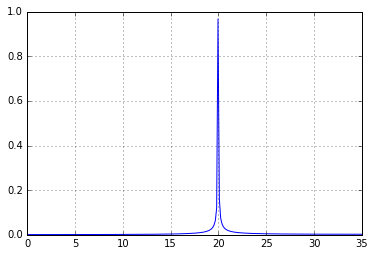Wow…the main frequency is $20$Hz… But $60-50$ is 10 and $70-50$ is 20!!
In :
# sample spacing
sampling=80
nsample = 500
dt = 1.0 / sampling
x = np.linspace(0.0, nsample*dt,nsample)
nu=50.0# frequency in Hz of the sine function
y = np.sin(nu * 2.0*np.pi*x)
yf = fft(y)
#xf = np.linspace(0.0, 1.0/(2.0*dt), nsample/2)
xf = np.linspace(0.0, 1.0/(2.0*dt), nsample/2)
plt.plot(x, (y[0:nsample]))
plt.grid()
plt.show()
plt.plot(xf, 2.0/nsample * np.abs(yf[0:nsample/2]))
plt.grid()
plt.show()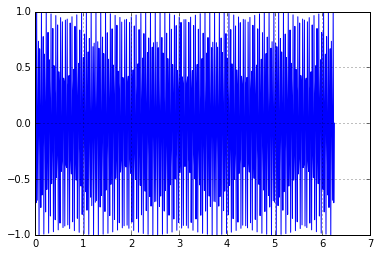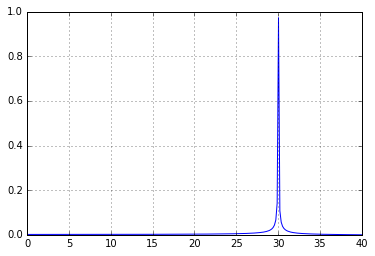If we use $sampling=80$Hz, again the main frequency is $sampling-nu=30$Hz
This is not a strange trick, this is the phenomenon of Aliasing, Wavy will afford in a next post.

#### Frequency resolution

Now, Wavy, I know you are thinking to your holiday,but we should answer also to the first question:
• What happen if we use longer nsample?
Let’s play now with the length of sample.
In :
# sample spacing
sampling=1024
nsample = 1000
dt = 1.0 / sampling
x = np.linspace(0.0, nsample*dt,nsample)
nu=50.0# frequency in Hz of the sine function
y = np.sin(nu * 2.0*np.pi*x)
yf = fft(y)
xf = np.linspace(0.0, 1.0/(2.0*dt), nsample/2)
plt.plot(x, (y[0:nsample]))
plt.grid()
plt.show()
plt.plot(xf, 2.0/nsample * np.abs(yf[0:nsample/2]))
plt.grid()
plt.show()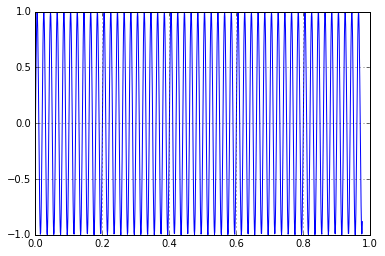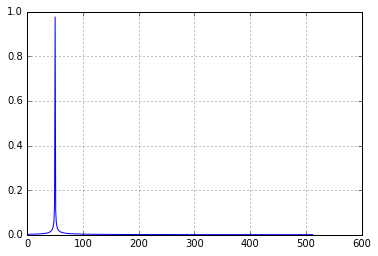In :
# sample spacing
sampling=1024
nsample = 10000
dt = 1.0 / sampling
x = np.linspace(0.0, nsample*dt,nsample)
nu=50.0# frequency in Hz of the sine function
y = np.sin(nu * 2.0*np.pi*x)
yf = fft(y)
xf = np.linspace(0.0, 1.0/(2.0*dt), nsample/2)
plt.plot(x, (y[0:nsample]))
plt.grid()
plt.show()
plt.plot(xf, 2.0/nsample * np.abs(yf[0:nsample/2]))
plt.grid()
plt.show()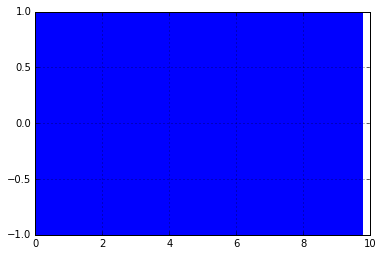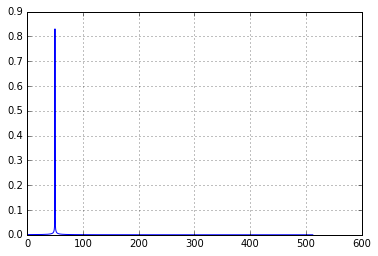We used in the first plot nsample=1000 and in the second plot nsample=10000.What we can see in the plots above is that if we use longer number of sample, our frequency resolution becomes better and better, that is the peak is more narrow. This is due to the relation between the 2 variables: the sampling frequency and the length of our FFT. Our bin resolution is just:$\displaystyle \frac{F_s}{N}$where$\displaystyle {F_s}$ is the input signal’s sampling rate and N is the number of FFT points used. In conclusion, for a given sampling frequency, longer sample will result in a better resolution.You can fork, download the code at the  GitHub Repo